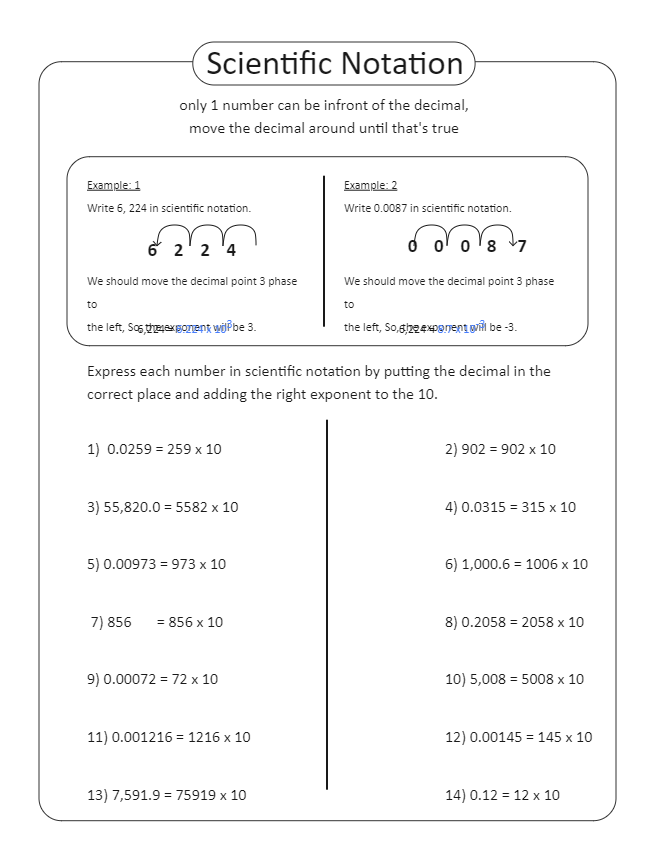Template Community / Scientific Notation Worksheet

# Scientific Notation Worksheet

Share
Duplicate0
0
Report
Publish time：02-10-2022
Scientific Notations are very important in research and development. In simpler words, scientific notation is writing very large or very small numbers. The scientific notation worksheet states how only one number can be in front of the decimal, and the student has to move the decimal until this becomes true. For example, if one has to write 0.0087 in the scientific notation, then as per the worksheet here, they must move the decimal point three places to the right. Then the exponent will be -3, and the term would become 8.7*10^-3.
CreatorKiraaaaPost
Recommended TemplatesPublish
Your
Diagram